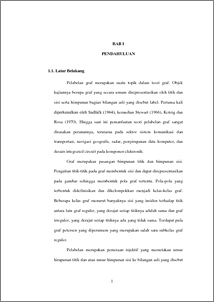# Vertex Magic Total Labeling Of Generalized Petersen Graphs

Rosyid, Abdul and Ratnasari , Lucia and Djuwandi, Djuwandi (2009) Vertex Magic Total Labeling Of Generalized Petersen Graphs. Undergraduate thesis, Universitas Diponegoro.Preview
PDF
277Kb

## Abstract

Let G = (V,E) be a simple and finite graph with a vertex – set V and an adge – set E. A vertex-magic total labeling of a graph G is a bijective mapping from V  E to {1, 2, 3, …, h} such that for each vertex x in Graph G satisfying λx+λ(xy)= k, where the sum is over all vertices y adjacent to x, for a constant k. Then k named a magic constant and G named vertex-magic total graph. In this paper we consider a vertex-magic labeling of generalized Petersen graph. The main focus is modeling of vertex-magic total labeling generalized Petersen graph and two copies of generalized Petersen graphs with a constant k.

Item Type: Thesis (Undergraduate) Q Science > QA Mathematics Faculty of Science and Mathematics > Department of Mathematics 2836 INVALID USER 21 Dec 2009 11:31 21 Dec 2009 11:31

Repository Staff Only: item control page